# Transient Natural Convection in Partially Porous Vertical Annuli

Transient Natural Convection in Partially Porous Vertical Annuli

F. Arpino A. Carotenuto M. Ciccolella G. Cortellessa N. Massarotti* A. Mauro

Dipartimento di Ingegneria Civile e Meccanica, Università di Cassino e del Lazio Meridionale, Via G. Di Biasio 43, 03043 Cassino (FR), Italy

Dipartimento di Ingegneria, Università degli Studi di Napoli “Parthenope”, Centro Direzionale, Isola C4, 80143 Napoli, Italy

Università Telematica Pegaso, Piazza Trieste e Trento n.48, 80132 Napoli, Italy

Corresponding Author Email:
massarotti@uniparthenope.it
Page:
S512-S518
|
DOI:
https://doi.org/10.18280/ijht.34S245
|
Accepted:
|
Published:
31 October 2016
| Citation

OPEN ACCESS

Abstract:

The numerical modeling of natural convection in partially porous annuli is a topic of rising interest, due to its practical applications for the provisional analyses of several engineering applications such as geothermal down-hole heat exchangers, chemical reactors, etc. Though a large number of papers focused on the modeling of this phenomenon under steady-state conditions, only few works consider its transient thermal evolution and no works are available referred to partially porous annular cavities. However, most of the real technical applications operate in dynamic mode, for this reason, this paper focuses on the transient numerical analysis of heat transfer in partially porous annular enclosures, where natural convection occurs. A fully explicit version of the CBS algorithm is employed here to solve the generalized porous medium model which describes both the sub-domains of the cavity using only one equations set; a dual time stepping technique is employed for transient analyses. Several cases have been analyzed, by changing the cavity aspect ratio and thermo-physical parameters of the fluid. Several results have been presented by changing both porous medium's properties and geometrical characteristics of the domain. The obtained results highlight that both the properties and the position of porous layer, inserted into the computational domain, strongly influence the thermal behavior of the cavity.

Keywords:

FEM, Porous medium model, Heat transfer, Dual time stepping.

1. Introduction

A better understanding of the convective processes occurring in partially porous enclosures is fundamental for the proper design of several real life applications involving the interaction between a porous matrix and a free fluid that saturates it [1-6]. Although scientific literature provides a large set of both experimental [7-9] and numerical data [10-13] for stationary free convection in vertical porous annuli, only few papers focus on partially porous annuli. Moreover, all these works are based on simplifying hypothesis; in fact, only few works referring to square cavities  or infinite domains  refer to very specific conditions, such as non-Boussinesq convection  or non-Newtonian viscoelastic fluids .   As regards thermal analysis of partially porous cavities, while laminar free convection in partially porous square and rectangular domains has been extensively analyzed both in steady [16-20] and transient conditions [21-23], few works concerning natural convection in partially vertical cylindrical cavities are available in scientific literature [24-29]. Moreover, only few papers [28-29] did not overlook transient regime, despite many engineering applications of the enclosure problem are related to real systems operating in dynamic mode. In order to improve the knowledge of the transient behavior of vertical partially porous annuli where buoyancy-driven heat and fluid flow occur, the generalized porous medium model has been solved applying an operator-splitting technique, the Artificial Compressibility (AC)- Characteristic-Based Split (CBS) algorithm, based on Characteristic Galerkin approach. Several analyses have been conducted by changing thermo-physical and geometrical features of two sub-domains to investigate the dependence of free convection on the cavity geometry.

2. Mathematical Model

For thermo-fluid analysis of problems involving the interaction between a layer assimilable to a porous medium and a free fluid which saturates it, two different approaches can be followed. A first one, called two domains approach, consists in solving two different equations for porous region and free fluid domain by defining properly coupling conditions for the interface. The formulation of these boundary conditions at the porous region- free fluid interface is not simple as often the different sub-regions are described by differential equations of different orders, e.g. Navier-Stokes and Darcy’s equation. Alternatively, a second approach more general can be introduced by defining a mathematical model based on a single equation suitable for application for both fluid and porous region. This model, called generalized porous medium model, is based on the concept of Representative Elementary Volume, whose size should be so that the values of the quantities of interest are independent of it; by averaging Navier-Stokes equations over this elementary unit using the volume averaging procedure introduced by Whitaker [30-31] it is possible to derive a generalized model that allows to define a single computational domain with different properties and therefore only matching conditions must be assumed at the interface, and there is no need for any empirical coefficient. Considering a uniform porous medium, under the hypothesis of Local Thermal Equilibrium, the non-dimensional form of the generalized model in cylindrical coordinates for the transient natural convection can be postulated as:

$\frac{1}{r} \frac{\partial\left(r \cdot u_{r}\right)}{\partial r}+\frac{\partial u_{z}}{\partial z}=0$(1)

$\frac{1}{\varepsilon} \frac{\partial u_{r}}{\partial t}+\frac{1}{\varepsilon^{2}}\left(u_{r} \frac{\partial u_{r}}{\partial r}+u_{z} \frac{\partial u_{r}}{\partial z}\right)=-\frac{\partial p_{f}}{\partial r}+$$\sqrt{\frac{\operatorname{Pr}}{R a}} \frac{J}{\varepsilon}\left[\frac{1}{r} \frac{\partial}{\partial r}\left(r \frac{\partial u_{r}}{\partial r}\right)-\frac{u_{r}}{r^{2}}+\frac{\partial^{2} u_{r}}{\partial z^{2}}\right]-\left(\frac{1}{D a} \sqrt{\frac{\operatorname{Pr}}{R a}}+\frac{F o}{D a}|\vec{u}|\right) u_{r}(2) \frac{1}{\varepsilon} \frac{\partial u_{z}}{\partial t}+\frac{1}{\varepsilon^{2}}\left(u_{r} \frac{\partial u_{z}}{\partial r}+u_{z} \frac{\partial u_{z}}{\partial z}\right)=-\frac{\partial p_{f}}{\partial z}+$$\sqrt{\frac{\operatorname{Pr}}{R a}} \frac{J}{\varepsilon}\left[\frac{1}{r} \frac{\partial}{\partial r}\left(r \frac{\partial u_{z}}{\partial r}\right)+\frac{\partial^{2} u_{z}}{\partial z^{2}}\right]-\left(\frac{1}{D a} \sqrt{\frac{\operatorname{Pr}}{R a}}+\frac{F o}{D a}\right) u_{z}+T$(3)

$\sigma \frac{\partial T}{\partial t}+u_{r} \frac{\partial T}{\partial r}+u_{z} \frac{\partial T}{\partial z}=\frac{\lambda}{\sqrt{\operatorname{Ra} \operatorname{Pr}}}\left(\frac{1}{r} \frac{\partial}{\partial r}\left(\frac{\partial(r \cdot T)}{\partial r}\right)+\frac{\partial^{2} T}{\partial y^{2}}\right)$(4)

Here, The Boussinesq approximation is introduced to model buoyancy effects.

The scales and parameters used in the non-dimensional form are:

\begin{align} & r=\frac{{{r}^{*}}}{L}{{;}^{{}}}{{^{{}}}^{{}}}T=\frac{{{T}^{*}}-T_{m}^{*}}{T_{h}^{*}-T_{c}^{*}}{{;}^{{}}}{{^{{}}}^{{}}}T_{m}^{*}=\frac{T_{h}^{*}+T_{c}^{*}}{2}{{;}^{{}}}{{^{{}}}^{{}}} \\ & Ra=\frac{g{{B}_{f}}\left( T_{h}^{*}-T_{c}^{*} \right){{L}^{3}}}{{{\nu }_{f}}{{\alpha }_{f}}}{{;}^{{}}}{{^{{}}}^{{}}}\Pr =\frac{{{\nu }_{f}}}{{{\alpha }_{f}}}; \\ & t={{t}^{*}}\frac{\sqrt{g\beta L\Delta T}}{L}{{;}^{{}}}{{^{{}}}^{{}}}p=\frac{{{p}^{*}}}{\rho g\beta L\Delta T}{{;}^{{}}}{{^{{}}}^{{}}}J=\frac{{{\mu }_{eff}}}{{{\mu }_{f}}}{{;}^{{}}}{{^{{}}}^{{}}} \\ & \lambda =\frac{{{\lambda }_{eff}}}{{{\lambda }_{f}}}{{;}^{{}}}{{^{{}}}^{{}}}Da=\frac{\kappa }{{{L}^{2}}};\sigma =\frac{\varepsilon {{\left( \rho {{c}_{p}} \right)}_{f}}+\left( 1-\varepsilon \right){{\left( \rho {{c}_{p}} \right)}_{s}}}{{{\left( \rho {{c}_{p}} \right)}_{f}}}{{;}^{{}}}{{^{{}}}^{{}}} \\ & {{\nu }_{f}}=\frac{{{\mu }_{f}}}{{{\rho }_{f}}}{{;}^{{}}}{{^{{}}}^{{}}}{{\alpha }_{f}}=\frac{{{\lambda }_{f}}}{{{\left( \rho {{c}_{p}} \right)}_{f}}}{{;}^{{}}}{{^{{}}}^{{}}}{{u}_{i}}=\frac{u_{i}^{*}}{\sqrt{g\beta L\Delta T}} \\ \end{align} (5)

The Navier-Stokes equations can be recovered by just setting $\varepsilon \rightarrow 1$ and $D a \rightarrow \infty$ in the above generalized model equations, while for $\varepsilon \rightarrow 0$ and $D a \rightarrow 0$ Eq. (4) describes the pure heat conduction through a solid. As the generalized model allows to simplify the numerical solution of highly coupled problems it is particularly suitable for the analysis of real life interface problems.

3. Numerical Model

The transient version of AC-CBS algorithm has been employed for the numerical solution of the PDEs constituting the generalized porous medium model. The governing equations were discretized in time along the flow characteristics, and in space using the standard Galerkin procedure

CBS-algorithm is based on a Characteristic Galerkin method, which stabilizes the oscillations due to convective terms by introducing higher-order convective terms with a temporal discretization along the characteristics and on a projection strategy which originates separate steps in the solution algorithm, allowing pressure stabilization. This method introduces a real time term into the fictitious momentum equations and into energy equation in order to progress in real time. So this technique implies the use of two time steps. The first is a “real” or outer or global or physical time step that coincides with the real physical time increment. Another is an artificial or inner or “local” or pseudo time step which is used to iterate the solution to gain each instantaneous steady-state . A steady state convergence criterion is set within each real time step; this means that the transient solution is calculated as temporal evolution of several pseudo-steady state levels.

Spatial discretization of the semi-discrete form of the algorithm is derived by applying the standard Galerkin finite element method with linear triangular prismatic elements. For the fully discretized form of Eqs. (1)-(4) reader can refer to . As the transient AC-CBS scheme presented here, employs a dual-time stepping technique in which each steady-state solution is derived with a fully explicit pseudo-time iterative procedure, an appropriate stability analysis is crucial for the definition of proper time-step values to avoid spurious oscillations. The proposed algorithm has been stabilized by employing an order of magnitude analysis of the different terms of the semi-discretized form of governing equations. Details on this are available in ref. . The code has been successfully validated through a comparison with experimental data available in scientific literature for steady state and transient natural convection in porous cylindrical domain; details about code validation can be found in ref. .

4. Results

In this section, the numerical results obtained for unsteady free convection in partially porous tall annuli with ARs of 4:1 and 8:1 are presented. In order to investigate the influence of porous layer position on thermo-fluid behavior of free convection in a partially porous vertical annulus, several analyses have been carried out by changing the position of the porous insert. In particular, two different configurations have been analyzed: a first one with the porous layer is on the left side of the computational domain (case A), and a second one obtained by exchanging the sub-domains (case B). The computational domain and the reference boundary conditions are represented in Figure 1. In particular, no slip conditions are assumed for all the walls of the cavity. The horizontal walls are assumed to be adiabatic, while Dirichlet temperature boundary conditions are imposed on vertical ones: in particular, the inner vertical boundary is kept at a dimensionless temperature Th=0.5 and the outer wall is at a fixed dimensionless temperature Tc=-0.5. Zero temperature and velocity fields are assumed as initial conditions. Moreover, the fluid is assumed to be incompressible and Newtonian, with a Prandtl number equal to 0.71, and the porous matrix is assumed to be isotropic and homogeneous, with a uniform porosity equal to 0.5.

## figure_1.png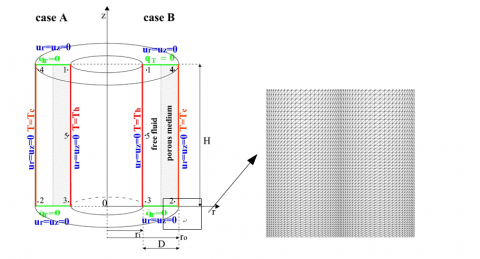Figure 1. Computational domain with a detail of computational mesh and boundary conditions employed

Figure 1 also shows a detail of the computational mesh used for the 4:1 cavity: it is composed of 14, 478 elements and 7, 679 nodes, while the mesh selected for the taller annulus consists of 30, 054 elements and 15, 592 nodes. Both grids are refined near all the boundaries of the computational domain and have been chosen after a mesh sensitivity analysis. In order to analyze the transient behavior of the natural convection, five points have been identified to analyze time variation of temperature: these points are represented in Figure 1 and their coordinates are reported in Table 1.

Table 1.   Non dimensional coordinates of probe points

 4:1 cavity 8:1 cavity Point r Z R z 1 ri + 0.091 3.685 ri + 0.181 7.370 2 ri + 0.910 0.315 ri + 0.819 0.630 3 ri + 0.091 0.315 ri + 0.181 0.630 4 ri + 0.910 3.685 ri + 0.819 7.370 5 ri + 0.091 2.00 ri + 0.181 4.00

Notes: see Figure 1.

The numerical investigations have been carried out assuming the value of Rayleigh number at which oscillations start both in the case of free fluid in a partially porous tall rectangular enclosure  (3.4 ∙ 106 for the 4:1 cavity and 3.4 ∙ 105 for the 8:1 cavity).

Temperature contours at real time t=500 are shown in Figures 2-5; in particular, Figure 2 and 3 refer to 4:1 annulus while the others refer to the taller cavity. A comparison between the temperature fields related to case (B) with that obtained for the case (A) shows that the position of the porous layer strongly affects the thermal behavior of the cavity: in fact, for both cases the configuration (B) leads to a chaotic evolution of temperature contours for high value of Da numbers whereas for very low values of Da, the heat conduction becomes dominant in both porous and free fluid region with positive effects on heat transfer.

## figure_2.png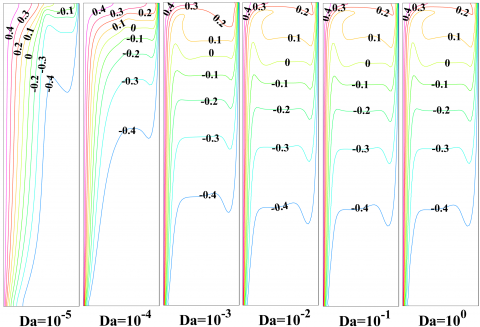Figure 2. Isotherms in a 4:1 partially porous annulus (ro/ri=2) for different Da and Ra=3.4 ∙ 106 (case A)

## figure_3.png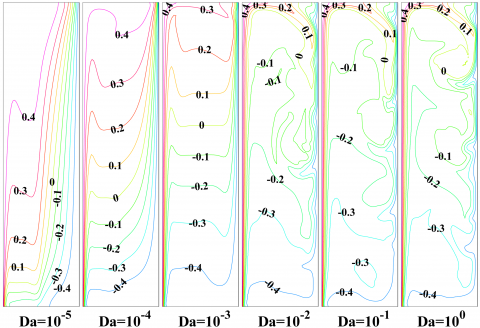Figure 3. Isotherms in a 4:1 partially porous annulus (ro/ri=2) for different Da and Ra=3.4 ∙ 106 (case B)

## figure_4.png## figure_4.png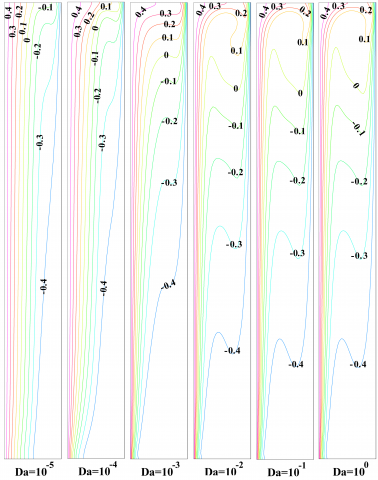Figure 4. Isotherms in a 8:1 partially porous annulus (ro/ri=2) for different Da and Ra=3.4 ∙ 105 (case A)

## figure_5.pngFigure 5. Isotherms in a 8:1 partially porous annulus (ro/ri=2) for different Da and Ra=3.4 ∙ 105 (case B)

Transient oscillations for two cases are quantitatively represented in Figures 6-7; in particular, Figure 6 refers to transient thermal behavior for different values of Da numbers for the shorter annulus while Figure 7 represents the same results for the taller cavity. The analysis of the results highlights that for high values of Da the presence of the porous layer does not damp non-periodic oscillations in the configuration B, whereas transient oscillations tend to disappear approaching steady state for the case A.

## figure_6.pngFigure 6. 4:1 annulus: Temperature time evolution at five probe points (see Table 1) for case A and case B for different Da and Ra=3.4 ∙ 106 (ro/ri=2)

## figure_7.png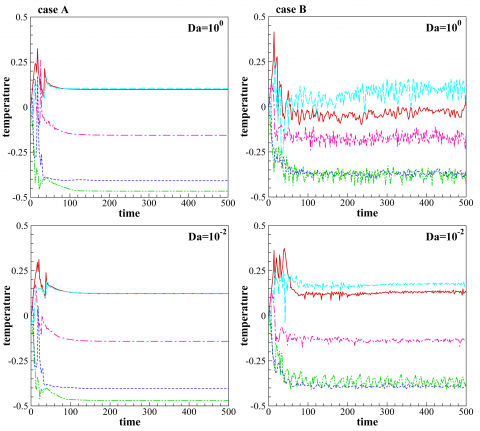Figure 7. 8:1 annulus: Temperature time evolution at five probe points (see Table 1) for case A and case B for different Da and Ra=3.4 ∙ 105 (ro/ri=2)

In order to investigate the behavior of the flow regime with the annulus radius, several analyses have been carried out by changing the radius ratio ro/ri in the range between 3 and 1.1.

As transient oscillations affect natural convection for high value of Darcy number, the reference value of Da=1 has been assumed for all the simulations. Figures 8 and 9 show the results obtained both for the case A and B for the two ARs.

## figure_8.png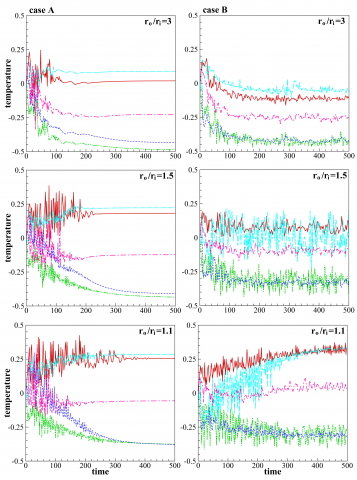Figure 8. 4:1 annulus: Temperature time evolution at five probe points (see Table 1) for case A and case B for different ro/ri and Ra=3.4 ∙ 106 (Da=1)

## figure_9.pngFigure 9. 8:1 annulus: Temperature time evolution at five probe points (see Table 1) for case A and case B for different ro/ri and Ra=3.4 ∙ 105 (Da=1)

In the first case, for all values of ro/ri, oscillations dampen and the flow is not oscillatory in time on all the considered points. As the value of radii ratio decreases, it is possible to observe an increase of the time necessary to reach the steady state. A different trend is observed for the results related to case B as transient oscillations do not disappear for all the values of radii ratios examined here. Finally, it can be noted that in both cases, the amplitude of these oscillations increases with the decrease of the value of radii ratio. It is interesting to note that transient oscillations are more evident and chaotic for the taller cavity.

5. Conclusions

In this paper, a properly stabilized fully matrix-inversion free scheme, employing an adaptive local time stepping procedure, is introduced for the analysis of unsteady free convection in partially porous annular enclosures. Several analyses have been conducted by changing the properties of the porous medium and its position in the annulus. The effect of different values of Darcy number for the porous medium and of the diameter of the annulus have been considered. The results highlight that not only the properties of the porous layer, but also its position in the annulus strongly affect the stability of the free convection. In particular, in this work it is shown that, by changing the position of internal porous domain, heat exchanged in the annulus can be enhanced; only for low values of the Darcy number the stability appears to be enhanced in both cases.

Nomenclature
 AR Bf aspect ratio isobaric compressibility of fluid K-1 cp Da Fo specific heat, J ∙ kg-1∙ K-1 Darcy number Forchheimer coefficient g J p Pr r Ra t T $\overrightarrow{u}$ ui z gravitational acceleration, m∙s-2 viscosity ratio pressure, Pa Prandtl number radial coordinate, m Rayleigh number real time, s temperature, °C velocity vector, m∙ s-1 seepage velocity components, m∙ s-1 vertical coordinate Greek symbols α thermal diffusivity, m2∙ s-1 ε porosity κ λ intrinsic permeability, m2 thermal conductivity, W∙ m-1∙K-1 µ ν ρ dynamic viscosity, kg ∙ m-1∙s-1 kinematic viscosity, m2 ∙ s-1 density, kg ∙ m-3 Subscripts c eff f h r z cold effective fluid hot radial component vertical component Superscripts * dimensional quantities
References

 M. Sankar, Y. Park, J. M. Lopez and Y. Do, “Numerical study of natural convection in a vertical porous annulus with discrete heating,” Int. J. Heat Mass Transf., vol. 54, no. 7, pp. 1493–1505, 2011. DOI: 10.1016/j.ijheatmasstransfer.2010.11.043.

 M. A. Teamah, W. M. El-Maghlany and M.M.K. Dawood, “Numerical simulation of laminar forced convection in horizontal pipe partially or completely filled with porous material,” Int. J. Therm. Sci., vol. 50, no. 8, pp. 1512–1522, 2011. DOI: 10.1016/j.ijthermalsci.2011.03.003.

 A. Carotenuto, N. Massarotti and A. Mauro, “A new methodology for numerical simulation of geothermal down-hole heat exchangers,” Appl. Therm. Eng., vol. 48, pp. 225–236, 2012. DOI: 10.1016/j.applthermaleng.2012.04.021.

 A. Mauro, F. Arpino, N. Massarotti and P. Nithiarasu, “A novel single domain approach for numerical modelling solid oxide fuel cells,” Int. J. Numer. Meth. Heat & Fluid Flow, vol. 20, no. 5, pp. 587-612, 2010. DOI: 10.1080/10407782.2013.756718.

 A. Mauro, F. Arpino and N. Massarotti, “Three-dimensional simulation of heat and mass transport phenomena in planar SOFCs,” Int. J. Hydrogen Energ, vol. 36, no. 16, pp. 10288-10301, 2011. DOI: 10.1016/j.ijhydene.2010.10.023.

 N. Massarotti, P. Nithiarasu and A. Carotenuto, “Microscopic and macroscopic approach for natural convection in enclosures filled with fluid saturated porous medium,” Int. J. Numer. Meth. Heat & Fluid Flow, vol. 13, no. 7, pp. 862-886, 2003. DOI: http://dx.doi.org/10.1108/09615530310502073.

 D. C. Reda, “Natural convection experiments in a liquid-saturated porous medium bounded by vertical coaxial cylinders,” ASME J. Heat Transf., vol. 105, no. 4, pp. 795–802, 1983. DOI: 10.1115/1.3246987.

 V. Prasad, F. A. Kulacki and M. Keyhani, “Natural convection in porous media,” J. Fluid Mech., vol. 150, pp. 89–119, 1985. DOI: 10.1017/s0022112085000040.

 V. Prasad, F. A. Kulacki and A. V. Kulkarni, “Free convection in a vertical porous annulus with constant heat flux on the inner wall-experimental results,” Int. J. Heat & Mass Transf., vol. 29, no. 5, pp. 713–723, 1986. DOI: 10.1016/0017-9310(86)90123-7.

 V. Prasad and F. A. Kulacki, “Natural convection in porous media bounded by short concentric vertical cylinders,” J. Heat Transf., vol. 107, no. 1, pp. 147–154, 1985. DOI: 10.1115/1.3247371.

 V. Prasad, “Numerical study of natural convection in a vertical, porous annulus with constant heat flux on the inner wall,” Int. J. Heat & Mass Transf., vol. 29, no. 6, pp. 841–853, 1986. DOI: 10.1016/0017-9310(86)90180-8.

 M. Hasnaoui, P. Vasseur, E. Bilgen and L. Robillard, “Analytical and numerical study of natural convection heat transfer in a vertical porous annulus,” Chem. Eng. Commun., vol. 131, no. 1, pp. 141–159, 1995. DOI: 10.1080/00986449508936288.

 I.A. Badruddin, Z. A. Zainal, Z. A. Kahn and Z. Mallick, “Effect of viscous dissipation and radiation on natural convection in a porous medium embedded within vertical annulus,” Int. J. Therm. Sci., vol. 46, no. 3, pp. 221-227, 2007. DOI: 10.1016/j.ijthermalsci.2006.05.005.

 A. M. Osman and H.M. Duwairi, “Forchheimer, non-Boussinseq natural convection in porous media filled enclosure”, Int. J. Heat Tech., vol. 33, no. 4, pp. 191-196, 2015. DOI: 10.18280/ijht.330425.

 K. Thirumurugan and R. Vasanthakumari, “Double-Diffusive convection of Non-Newtonian Walters' (MODELB') viscoelastic fluid through porous medium with suspended particles”, Int. J. Heat Tech., vol. 34, no. 3, pp. 357-363, 2016. DOI: 10.18280/ijht.340302.

 T. W. Tong and E. Subramanian, “Natural convection in rectangular enclosures partially filled with a porous medium,” Int. J. Heat & Fluid Flow, vol. 7, no. 1, pp. 3-10, 1986. DOI: 0.1016/0142-727x(86)90033-0.

 C. Beckermann, S. Ramadhyani and R. Viskanta, “Natural convection flow and heat transfer between a fluid layer and a porous layer inside a rectangular enclosure,” ASME J. Heat Transf., vol. 109, no. 2, pp. 363-370, 1987. DOI: 10.1115/1.3248089.

 M. Song and R. Viskanta, “Natural convection flow and heat transfer within a rectangular enclosure containing a vertical porous layer,” Int. J. Heat & Mass Transf., vol. 37, no. 16, pp. 2425-2438, 1994. DOI: 10.1016/0017-9310(94)90284-4.

 A. K. Singh and G. R. Thorpe, “Natural convection in a confined fluid overlying a porous layer – a comparison study of different models,” Indian J. pure ap. Mat., vol. 26, pp. 81-95, 1995. http://www.new1.dli.ernet.in/data1/upload/insa/INSA_2/20005a1a_81.pdf

 T. Paul, B. K. Jha and A. K. Singh, “Free convection between vertical walls partially field with porous medium,” Heat Mass Transf., vol. 33, no. 5-6, pp. 515–519, 1998. DOI: 10.1007/s002310050223.

 A. K. Singh, P. Agnihotri, N. P. Singh and A. K. Singh, “Transient and non-Darcian effects on natural convection flow in a vertical channel partially filled with porous medium: analysis with Forchheimer-Brinkman extended Darcy model,” Int. J. Heat & Mass Transf., vol. 54, no. 5, pp. 1111-1120, 2011. DOI: 10.1016/j.ijheatmasstransfer.2010.11.011.

 S.-G. Yao, L.-B. Duan, Z.-S. Ma and X.-W. Jia, “The study of natural convection heat transfer in a partially porous cavity based on LBM,” The Open Fuels & Energ. Sci. J., vol. 7, pp. 88-93, 2014. http://bentham-open.com/contents/pdf/TOEFJ/TOEFJ-7-88.pdf

 F. Arpino, G. Cortellessa and A. Mauro, “Transient thermal analysis of natural convection in porous and partially porous cavities,” Numer. Heat Transf., A: Appl., vol. 67, no. 6, pp. 605-631, 2015. DOI: 10.1080/10407782.2014.949133.

 S. Kiwan and M. S. Al-Zahrani, “Effect of using porous inserts on natural convection heat transfer between two concentric vertical cylinders,” Numer. Heat Transf., A: Appl., vol. 53, no. 8, pp. 870–889, 2008. DOI: 10.1080/10407780701715869.

 M. Benzeghiba, S. Chikh and A. Campo, “Thermosolutal convection in a partly porous vertical annular cavity,” ASME J. Heat Transf., vol. 125, no. 4, pp. 703–715, den2003. DOI: 10.1115/1.1589501.

 P. Venkata Reddy and G.S.V.L. Narasimham, “Natural convection in a vertical annulus driven by a central heat generating rod,” Int. J. Heat & Mass Transf., vol. 51, no. 19, pp. 5024–5032, 2008. DOI: 10.1016/j.ijheatmasstransfer.2008.02.032.

 F. Arpino, A. Carotenuto, N. Massarotti and A. Mauro, “New solutions for axial flow convection in porous and partly porous cylindrical domains,” Int. J. Heat & Mass Transf., vol. 57, no. 1, pp. 155-170, 2013. DOI: 10.1016/j.ijheatmasstransfer.2012.10.030.

 M. A. Sheremet and T. A. Trifonova, “Unsteady Conjugate Natural Convection in a Vertical Cylinder Partially Filled with a Porous Medium,” Numer. Heat Transf., A: Appl., vol. 64, no. 12, pp. 994-1015, 2013. DOI: 10.1080/10407782.2013.811973.

 M. Ciccolella, G. Cortellessa, N. Massarotti and A. Mauro, “New benchmark solutions for transient natural convection in partially porous annuli,” Int. J. Numer. Meth. Heat & Fluid Flow, vol. 26, no. 3-4, 2016. http://www.emeraldinsight.com/doi/pdfplus/10.1108/HFF-11-2015-0464

 S. Whitaker, “Diffusion and Dispersion in Porous Media,” AIChE J., vol. 13, no. 3, pp. 420–427, 1961. DOI: 10.1002/aic.690130308.

 C. T. Hsu and P. Cheng, “Thermal Dispersion in a Porous Medium,” Int. J. Heat & Mass Transf., vol. 33, no. 8, pp. 1589–1597, 1990. DOI: 10.1016/0017-9310(90)90015-M.

 F. Arpino, G. Cortellessa, M. Dell'Isola, N. Massarotti and A. Mauro, “High order explicit solutions for the transient natural convection of incompressible fluids in tall cavities,” Numer. Heat Transf., A: Appl., vol. 66, no. 8, pp. 839-862, 2014. DOI: 10.1080/10407782.2014.892389.